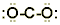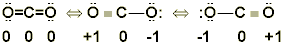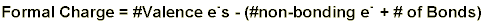Background The premise behind Lewis structures is the octet rule: that all atoms would like to be surrounded with an octet of electrons. Of course, there are, some exceptions: very small atoms (H, Be and B) have less than an octet, and some main group atoms with low energy d orbitals (P, S, Cl, Br, and I) may have more than an octet. This is especially true when these atoms are central atoms and combined with highly electronegative atoms. Drawing correct Lewis structures takes practice but the process can be simplified by following a series of steps:   Step 1.             Count all the valence electrons for each atom. Add or subtract electrons if  the structure is an anion or cation, respectively. Step 2.             Determine which atoms are bonded to one another. Draw a skeletal structure. Step 3.             Connect the atoms with a pair of electrons in each bond. Subtract the bonding electrons from the total valence electrons. Step 4.             Add electron pairs to complete octets for all peripheral atoms attached to the central atom. Beware of hydrogen – hydrogen never has more than one bond or one pair of electrons Step 5.             Place remaining electrons on the central atom, usually in pairs. The octet rule may be exceeded for P, S, Cl, Br, or I. Step 6.             If the central atom does not have an octet, form double or triple bonds by moving electron pairs from one or more peripheral atoms to achieve an octet. Step 7.             Look for resonance structures by rearranging bonds.  The structure with the lowest total formal charges will be the most likely form to be found in nature. (See below for explanation)                         Drawing Lewis Structures Let's look at an example of how this works using a real molecule. Consider the molecule most responsible for the greenhouse effect, carbon dioxide (CO2). To draw the Lewis structure: Step 1. Count all the valence electrons for each atom: Carbon 1 x 4 valence electrons = 4 electrons Oxygen 2 x 6 valence electrons = 12 electrons Total = 16 electrons Step 2. Determine which atoms are bonded to one another. Generally the least electronegative atom is the central atom. However if the only choice is between a more electronegative atom and hydrogen, the more electronegative atom will be the central atom (e.g. water). Hydrogen NEVER makes more than one bond and thus can NEVER be the central atom.   For CO2, carbon is the less electronegative atom so it should be the central atom.Step 3. Connect each atom with a single pair of electrons or single bond: (16 valence electrons – 4 bonding electrons  = 12 electrons left.)Step 4. Add electron pairs to peripheral atoms for octets:Step 5. No electrons are left over, but the central atom doesn’t have an octet! Step 6. Move electrons from peripheral atoms, forming double bonds to give the central atom an octet:Step 7. Look for resonance structures and identify the one with the smallest formal charges:For some molecules, more than one structure can be drawn. Note that a Lewis structure for carbon dioxide can be written using a carbon-oxygen single bond on one side and carbon-oxygen triple bond on the other. How can these two possibilities be distinguished? How can the most important structure be chosen, or are they all equally likely? When several structures can be drawn, they are called resonance structures. Resonance Structures In resonance structures, all the atoms are in the same relative position to one another, but the distribution of electrons around them is different. To evaluate the importance of each structure, the formal charge on each atom must be determined. Formal Charge Formal charge is a somewhat arbitrary way of describing how many electrons an atom seems to have in a particular compound. Electron pairs in bonds between atoms are assumed to be split equally between the two atoms. Non-bonding electron pairs are counted as belonging to the atom on which they reside. This can be put into an equation:orThe most stable resonance structure is the one in which: 1.         There is a minimum number of formal charges; 2.         If there are formal charges, like charges are separated; and 3.         Negative formal charges are on the more electronegative atoms and positive formal charges are on the less electronegative atoms. For the CO2 structure with two double bonds, the formal charges can be calculated as follows: Oxygens: Formal Charge = 6 - (4 + 1/2(4)) = 0 Carbon: Formal Charge = 4 - ( 0+ 1/2(8)) = 0 For the CO2 structure with a single and triple bond: Oxygen (single): Formal Charge = 6 - (6 + 1/2(2)) = -1 Oxygen (triple): Formal Charge = 6 - (4 + 1/2(6)) = +1 Carbon: Formal Charge = 4 - ( 0+ 1/2(8)) = 0 So, while both structures work as Lewis structures, the one which results in zero formal charges for any of the atoms is more stable and thus more likely to exist in nature than the one having charges on the two oxygen atoms. Oxidation Numbers Formal charges need to be distinguished from oxidation numbers (which can also be determined from Lewis structures). Oxidation numbers are used to indicate whether a molecule is neutral, electron-rich or electron-poor.  The rules for determining oxidation numbers are found in your textbook.  A short summary of these rules is given here: 1. The oxidation number for an element in its elemental form is 0 (holds true for isolated atoms and for molecular elements, e.g. Cl2 and P4.,) 2. The oxidation number of a monatomic ion is the same as its charge (e.g. oxidation number of Na+ = +1, and that of S2- is -2.) 3. In binary compounds the element with greater electronegativity is assigned a negative oxidation number equal to its charge if found in simple ionic compounds (e.g. in the compound PCl3 the chlorine is more electronegative than the phosphorus. In simple ionic compounds Cl has an ionic charge of 1-, so its oxidation state in PCl3 is -1) 4. The sum of the oxidation numbers is zero for an electrically neutral compound and equals the overall charge for an ionic species. 5. Alkali metals exhibit only an oxidation state of +1 in compounds. 6. Alkaline earth metals exhibit only an oxidation state of +2 in compounds.   Once Lewis structures are drawn successfully, they can be used to predict the electron cloud geometry, molecular shape and polarity of molecules and ions. For a thorough discussion, refer to your textbook. In particular, look at the 3-dimensional representations for all the geometries and shapes. Electron Cloud Geometry and Molecular Geometry The electron cloud geometry around a central atom is determined by the number of electron groups surrounding it. Each set (2, 3, 4, 5, and 6) has a different name and arrangement in three dimensional space.  Electron clouds, all being negative, are most stable when separated as far from one another as possible. This is called the valence shell electron pair repulsion theory (VSEPR). While electron cloud geometry describes the orientation of the electrons around the central atom, the molecular geometry describes the arrangement of peripheral atoms.  The Experiment In the lab you will be presented with six molecular models as unknowns. It will be your job to name them.  You will also be asked to determine their electron pair and molecular geometries by measuring their bond angles using a protractor.  A worksheet is provided containing other questions that should be completed for each of the molecules.  You should make five additional copies of the worksheet to use during class.  These worksheets will then be used as the data section of your lab report.